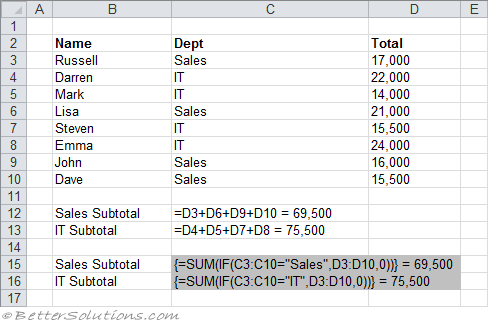# Sum cells that meet either condition

2) Getting subtotals [Using a single cell array formula]
This example illustrates two methods that can be used to obtain subtotals from a table of data.The formulas in cells C12 & C13 use the plus operator to sum the individual cells.
Entering these manually would be quite time consuming if the list was any longer. Each cell must be added manually to the formula.
The formulas in cells C15 & C16 use an array formula to add up the subtotals and must be entered using (Ctrl + Shift + Enter), otherwise #VALUE! will be returned.
These array formulas use two 1-dimensional arrays, C3:C10 and D3:D10.
The IF function compares the corresponding values in the first array C3:C10 and then returns the corresponding value from the second array D3:D10.
The SUM function is then passed this new array (which exists only in memory) and returns the total value.